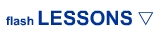ADVANCED/GAME Lesson 1: Control Movieclips Lesson 2: Flip Symbol Lesson 3: Inside Movieclip Lesson 4: Dynamic Text Lesson 5: Random Clip Lesson 6: Actionscript Music Lesson 7: Symbol Timer Lesson 8: Rotate Movieclip Lesson 9: Disable Right-click Lesson 10: Frame Names Lesson 11: Side Scroller Lesson 12: Increasing Velocity Lesson 13: Visibility Lesson 14: What's 'this' Lesson 15: Collectable Items Lesson 16: Specified Action Lesson 17: Button Tap Code Lesson 18: Random in Detail Lesson 19: Health Bar Lesson 20: Follow Movieclips MORE GAMES Lesson 1: Movement Lesson 2: Wall Collision Lesson 3: Shoot Lesson 4: Shot Collision Lesson 5: Score ANIMATION IN FLASH Lesson 1: Character Lesson 2: Eye Movement Lesson 3: Arm Movement Lesson 4: Walking Lesson 5: Background Lesson 6: Loop Background ROB's CORNER Introduction Lesson 1: Orbital Dots 1 Lesson 2: Orbital Dots 2 Lesson 3: Spinning 1 Lesson 4: Spinning 2 Lesson 5: Easing Spaceship Lesson 6: Sprite 3d Lesson 7: Bouncy Ball Lesson 8: Bouncy Ball 2 2 Lesson 9: Simple Collision Lesson 10: Crazy Tiles ****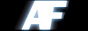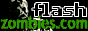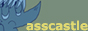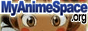Link Us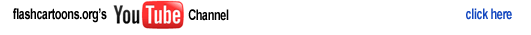home > learn flash > actionscript 3.0 lesson 9

Actionscript 3.0 Lesson Nine: Adding A Timer

 1.) In this tutorial I will show you how to create a timer. To keep things consistant I will provide an example of by puting a textbox on the stage and giving it an instance name of "timeString". Then give it some variables and actions of how to increment the timer. The output will look like this Time: 1:35. var startTime:int = getTimer(); addEventListener(Event.ENTER_FRAME, showClock); function showClock(event:Event){ //ms passed var timePassed:int = getTimer()-startTime; //computer minutes and seconds var seconds:int = Math.floor(timePassed/1000); var minutes:int = Math.floor(seconds/60); seconds -= minutes*60; //convert clock to string var timeString:String = "Timer " + minutes + " : " + String(seconds + 100).substr(1,2); //show in text field' timeDisplay.text = timeString; } --------- -------------- --------- --------------2.) This code basically calls a couple functions and executes a few lines of code that does an equation of what needs to be done to make a propper game time string. -jordan